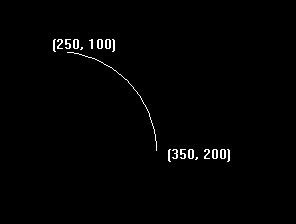# getarcoords() function in C

The header file graphics.h contains getarccoords() function which is used to get coordinates of arc which is drawn most recently. arccoordstype is a predefined structure which is defined as follows:

Syntax :

```struct arccoordstype
{
// center point of arc
int x, y;

// start position
int xstart, ystart;

// end position
int xend, yend;
};
```

Note : address of a structure variable of type arccoordstype is passed to function getarccoords.

Syntax :

```void getarccoords(struct arccoordstype *var);
```

Example :

```Input : x = 250, y = 200, s_angle = 0 ,
e_angle = 90, radius = 100
Output :```

Below is the implementation of getarccoords() function

 `// C Implementation for getarccoords() ` `#include ` `#include ` ` `  `// driver code ` `int` `main() ` `{ ` `    ``// gm is Graphics mode which is ` `    ``// a computer display mode that ` `    ``// generates image using pixels. ` `    ``// DETECT is a macro defined in ` `    ``// "graphics.h" header file ` `    ``int` `gd = DETECT, gm; ` ` `  `    ``// p is structure variable ` `    ``// of type arccoordstype ` `    ``struct` `arccoordstype p; ` `    ``char` `arr; ` ` `  `    ``// initgraph initializes the ` `    ``// graphics system by loading a ` `    ``// graphics driver from disk ` `    ``initgraph(&gd, &gm, ``""``); ` ` `  `    ``// arc function is used to draw an  ` `    ``// arc the first 2 arguments are  ` `    ``// center of arc  3rd and 4th  ` `    ``// arguments are starting and   ` `    ``// ending angles. Last argument  ` `    ``// is the radius. ` `    ``arc(250, 200, 0, 90, 100); ` ` `  `    ``// getarccoords function which takes ` `    ``// address of a structure variable of ` `    ``// type arccoordstype as an argument. ` `    ``getarccoords(&p); ` ` `  `    ``// sprintf stands for “String print”. ` `    ``// Instead of printing on console, it ` `    ``// store output on char buffer which ` `    ``// are specified in sprintf ` `    ``sprintf``(arr, ``"(%d, %d)"``, p.xstart,  ` `                             ``p.ystart); ` ` `  `    ``// outtext function displays text ` `    ``// at current position. ` `    ``outtextxy(360, 195, arr); ` ` `  `    ``sprintf``(arr, ``"(%d, %d)"``, p.xend,  ` `                            ``p.yend); ` ` `  `    ``outtextxy(245, 85, arr); ` ` `  `    ``getch(); ` ` `  `    ``// closegraph function closes the ` `    ``// graphics mode and deallocates ` `    ``// all memory allocated by ` `    ``// graphics system . ` `    ``closegraph(); ` ` `  `    ``return` `0; ` `} `

Output :

``````

My Personal Notes arrow_drop_upIn love with a semicolon because sometimes i miss it so badly)

If you like GeeksforGeeks and would like to contribute, you can also write an article using contribute.geeksforgeeks.org or mail your article to contribute@geeksforgeeks.org. See your article appearing on the GeeksforGeeks main page and help other Geeks.

Please Improve this article if you find anything incorrect by clicking on the "Improve Article" button below.

Article Tags :
Practice Tags :

Be the First to upvote.

Please write to us at contribute@geeksforgeeks.org to report any issue with the above content.